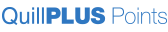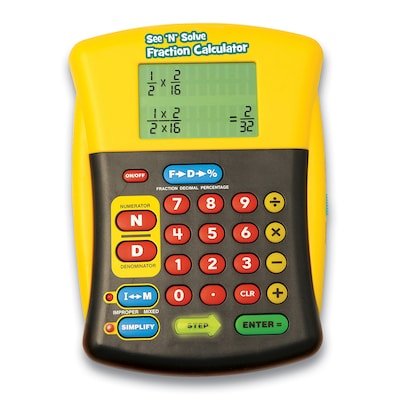Turn every order into a gift. Join Today!
Close

# Educational Insights See 'N’ Solve Fraction Calculator (EI-8479)

Item #: 901-JV36643
Model #: EI8479
Brand: Educational Insights\$31.99 Each
Delivery Date:

Enter Zip Code
Description
See 'n' solve fraction calculator helps in addition, subtraction, multiplication and division for grade 3+. The See 'n' Solve Fraction Calculator is the only user-friendly calculator on the market that shows students how to solve a fraction problem, step by step! The easy-to-use format reinforces introductory fraction skills without the complications of other scientific calculators - making it perfect for school and home. Students can add, subtract, multiply, and divide fractions easily. Press the special Numerator and Denominator keys to input a fraction problem. The large 2-line screen displays the problem (with common denominator), and lighted keys guide students through the steps to solve it. Special keys instantly simplify fractions or convert fractions to decimals or percentages. A terrific way for students to see and understand how to solve any fraction problem! Requires 2 AA batteries (not included).
• Age group and grade: Ages 6 - 11 and grades 3+
• Subject: Calculators
• Usage ideas: Easily input addition, subtraction, multiplication, division equations or convert between fractions, decimals, percents and also features normal calculator mode
• Size: 6 1/4"(H) x 4 1/2"(W)
• Includes 12 page activity guide and quick reference stickers
• Lighted keys guide you through the problem, press special keys to reduce a fraction or change it to a mixed number, an improper fraction, a decimal or percentage
• Helps in addition, subtraction, multiplication and division
• Let this unique calculator model solving fraction problems step-by-step
• Oversize LCD screen displays each step, shows amounts in mixed number and improper fraction form, and answers in reduced form
• The See ‘N’ Solve Fraction Calculator takes complicated fraction calculations and simplifies them!
• Easily adds, subtracts, multiplies, and divides fractions; simply press the Numerator and Denominator keys to input a fraction problem
• Large 2-line screen displays the problem and the steps to solve it
• A terrific way for students to see and understand how to solve any fraction problem!
• Requires 2 "AA" batteries (not included)
• User-friendly calculator that shows students how to solve a fraction problem, step by step
• Easy-to-use format reinforces introductory fraction skills-perfect for school and home
• Students can add, subtract, multiply, and divide fractions easily
• Press the special Numerator and Denominator keys to input a fraction problem
Specifications
 Brand : Educational Insights Buttons : Features 4 buttons (addition, subtraction, multiplication, division). Color Family : Yellow (33477) Customizable : No Height in Inches : 6.25 Power Source : Battery True Color : Multicolor Type of Display : LCD Weight : 0.80 lbs. per Each Width in Inches : 4.5 Weight : 0.80 lbs. per Each

### AccessoriesLoading...